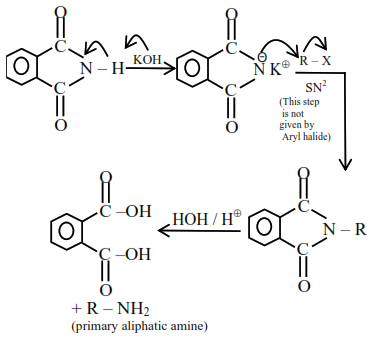# Given below are two statements,`
Question:

Given below are two statements, one is labelled as Assertion (A) and other is labelled as Reason (R).

Assertion (R) : Gabriel phthalimide synthesis cannot be used to prepare aromatic primary amines.

Reason : Aryl halides do not undergo nucleophilic substitution reaction.

In the light of the above statements, choose the correct answer from the options given below :

1. Both (A) and (R) true but (R) is not the correct explanation of (A).

2. (A) is false but $(R)$ is true.

3. Both (A) and (R) true and (R) is correct explanation of (A).

4. (A) is true but (R) is false.

Correct Option: , 3

Solution:

Gabriel pthalamide synthesis